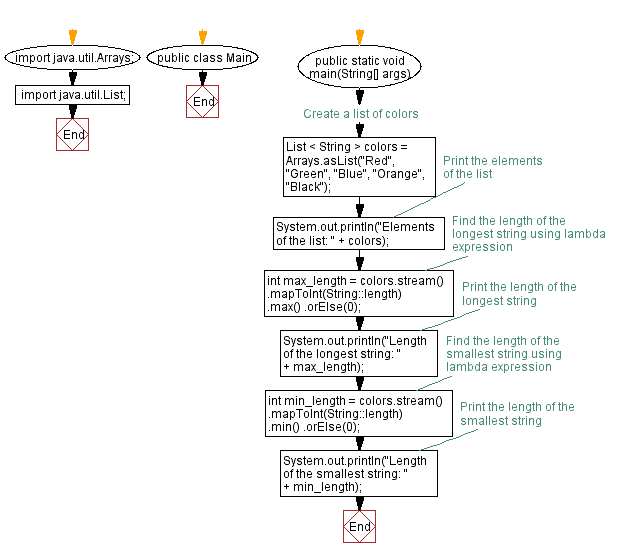# Java program to find length of longest and smallest string using lambda expression

## Java Lambda Program: Exercise-17 with Solution

Write a Java program to implement a lambda expression to find the length of the longest and smallest string in a list.

Sample Solution:

Java Code:

``````import java.util.Arrays;
import java.util.List;

public class Main {
public static void main(String[] args) {
// Create a list of colors
List < String > colors = Arrays.asList("Red", "Green", "Blue", "Orange", "Black");

// Print the elements of the list
System.out.println("Elements of the list: " + colors);

// Find the length of the longest string using lambda expression
int max_length = colors.stream()
.mapToInt(String::length)
.max()
.orElse(0);
// Print the length of the longest string
System.out.println("Length of the longest string: " + max_length);

// Find the length of the smallest string using lambda expression
int min_length = colors.stream()
.mapToInt(String::length)
.min()
.orElse(0);
// Print the length of the smallest string
System.out.println("Length of the smallest string: " + min_length);
}
}
``````

Sample Output:

```Elements of the list: [Red, Green, Blue, Orange, Black]
Length of the longest string: 6
Length of the smallest string: 3
```

Explanation:

In the above exercise,

• Import the necessary classes: Arrays and List.
• In the main method, we create a list of colors called colors using Arrays.asList().
• Use the stream() method on the colors list to create a stream of strings.
• Use the mapToInt() method to map each string to its integer length.
• The max() method is used to get the maximum length of a stream of integers.
• The min() method is used to get the minimum length among a stream of integers.
• The orElse() method is used to provide a default value of 0 in case the stream is empty.
• Finally, we print the length of the longest and smallest string.

Flowchart:Live Demo:

Java Code Editor:

Improve this sample solution and post your code through Disqus

Java Lambda Exercises Previous: Java program to check if a list contains a specific word using lambda expression.
Java Lambda Exercises Next: Java program to check if a number is a perfect square using lambda expression.

What is the difficulty level of this exercise?

Test your Programming skills with w3resource's quiz.

﻿

## Java: Tips of the Day

Capturing an exception's stack trace

```import java.io.*;

Exception e = ...;
java.io.StringWriter sw = new java.io.StringWriter();
e.printStackTrace(new java.io.PrintWriter(sw));
String trace = sw.getBuffer().toString();
```

Ref: https://bit.ly/3k8PmGm

We are closing our Disqus commenting system for some maintenanace issues. You may write to us at reach[at]yahoo[dot]com or visit us at Facebook